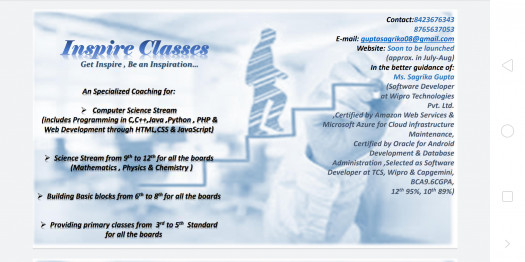# CBSE & Upb Class 10th-real Numbers Online Assessment

15 Questions | Total Attempts: 33SettingsCreate your own Quiz• 1.
Find the HCF of 1848, 3058 and 1331.
• A.

9

• B.

14

• C.

13

• D.

11

• 2.
Find the biggest number which can divide both 324 and 144.
• A.

18

• B.

36

• C.

9

• D.

21

• 3.
Write down the decimal expansions of :  13/ 6250
• A.

0.0208

• B.

0.00208

• C.

0.00512

• D.

0.00416

• 4.
Which of the following is rational?
• A.

(2-√3)^2

• B.

(√2 + √3)^2

• C.

(√2 -√3)(√2 + √3)

• D.

(2√7)/7

• 5.
The product of a rational and irrational number is:
• A.

Rational no.

• B.

Irrational no.

• C.

Both of the above

• D.

None of the above

• 6.
The sum of a rational and irrational number is:
• A.

Rational

• B.

Irrational

• C.

Both of the above

• D.

None of the above

• 7.
If b = 3, then any integer can be expressed as a =
• A.

3q, 3q+ 1, 3q + 2

• B.

3q

• C.

None of these

• D.

3q+1

• 8.
Express 98 as a product of its primes
• A.

2² × 7

• B.

2² × 7²

• C.

2 × 7²

• D.

None of these

• 9.
(6 + 5 √3) – (4 – 3 √3) is
• A.

A rational number

• B.

An irrational number

• C.

A natural number

• D.

An integer

• 10.
If HCF (16, y) = 8 and LCM (16, y) = 48, then the value of y is
• A.

24

• B.

16

• C.

8

• D.

48

• 11.
If LCM (77, 99) = 693, then HCF (77, 99) is
• A.

11

• B.

7

• C.

9

• D.

22

• 12.
The ratio between the LCM and HCF of 5, 15, 20 is:
• A.

9:1

• B.

4:3

• C.

11:1

• D.

12:1

• 13.
987/10500 will have
• A.

Terminating decimal expansion

• B.

Non terminating & Non repeating Decimal expansion

• C.

Non terminating repeating Decimal expansion

• D.

None of these

• 14.
A rational number between 1/2 and 3/4 is:
• A.

2/5

• B.

5/8

• C.

4/3

• D.

1/4

• 15.
The number π is:
• A.

A Natural Number

• B.

A Rational Number

• C.

An Irrational Number

• D.

A Whole Number

Related TopicsBack to top
×

Wait!
Here's an interesting quiz for you.